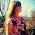MATH 1280 Introduction to Statistics – Midterm Exam – part 3

Caravaggio painting

Midterm Part 3 – question 7 only, include its answer (total questions is 16)

7) Load data for Group B into an R object called B, then run this command:
barplot(table(B))
> B<-c(9, 8, 7, 8, 9, 10, 9, 10, 7, 8, 10, 9, 9, 4, 6, 3)
> barplot(table(B)).

What does the bar on the right represent?
Select one:
a. Nine students received a score of 5 on the quiz.
b. One student received a score of 6 on the quiz.
c. Three students received a score of 7 on the quiz.
d. Four students received a score of 9 on the quiz.
e. Three students received a score of 10 on the quiz….> Correct

Another Caravaggio painting

# To be continued to part 4

1.Aaa-Haa I missed the lesson on "Greek" in high school. I was busy painting my nails while the teacher was teaching Greek. (((: HaHaHaaa

I'm just saying hi, stopping in to see what's new with you and to show my face. I hope you had a nice weekend.

Wishing you a wonderful week!

1.Thank you to visit:
MATH 1280 Introduction to Statistics – Midterm Exam – part 3
@
Caravaggio painting

Midterm Part 3 – question 7 only, include its answer (total questions is 16)

2.MATH 1280 Introduction to Statistics – Midterm Exam – part 3
-
What does the bar on the right represent?

Select one:
a. Nine students received a score of 5 on the quiz.
b. One student received a score of 6 on the quiz.
c. Three students received a score of 7 on the quiz.
d. Four students received a score of 9 on the quiz.
e. Three students received a score of 10 on the quiz….> Correct

2.I tried reading this.
I got a brain cramp.
Time for a beer.

1.Thank you to visit:
MATH 1280 Introduction to Statistics – Midterm Exam – part 3
@
7) Load data for Group B into an R object called B, then run this command:
barplot(table(B))
> B<-c(9, 8, 7, 8, 9, 10, 9, 10, 7, 8, 10, 9, 9, 4, 6, 3)
> barplot(table(B)).

2.MATH 1280 Introduction to Statistics – Midterm Exam – part 3
-
Midterm Part 3 – question 7 only, include its answer (total questions is 16)

7) Load data for Group B into an R object called B, then run this command:
barplot(table(B))
> B<-c(9, 8, 7, 8, 9, 10, 9, 10, 7, 8, 10, 9, 9, 4, 6, 3)
> barplot(table(B)).

3.MATH 1280 Introduction to Statistics – Midterm Exam – part 3

3.Muy bonito todo! Me encantaría verte por mi blog! Feliz día precios@! 💗💗💗

1.Thank you to visit:
MATH 1280 Introduction to Statistics – Midterm Exam – part 3
@
What does the bar on the right represent?

Select one:
a. Nine students received a score of 5 on the quiz.
b. One student received a score of 6 on the quiz.
c. Three students received a score of 7 on the quiz.
d. Four students received a score of 9 on the quiz.
e. Three students received a score of 10 on the quiz….> Correct

2.MATH 1280 Introduction to Statistics – Midterm Exam – part 3
-
What does the bar on the right represent?

Select one:
a. Nine students received a score of 5 on the quiz.
b. One student received a score of 6 on the quiz.
c. Three students received a score of 7 on the quiz.
d. Four students received a score of 9 on the quiz.
e. Three students received a score of 10 on the quiz….> Correct

4.Roaming saya mas

1.Thank you to visit:
MATH 1280 Introduction to Statistics – Midterm Exam – part 3
@
Midterm Part 3 – question 7 only, include its answer (total questions is 16)

7) Load data for Group B into an R object called B, then run this command:
barplot(table(B))
> B<-c(9, 8, 7, 8, 9, 10, 9, 10, 7, 8, 10, 9, 9, 4, 6, 3)
> barplot(table(B)).

2.MATH 1280 Introduction to Statistics – Midterm Exam – part 3
-
Another Caravaggio painting

# To be continued to part 4

3.MATH 1280 Introduction to Statistics – Midterm Exam – part 3

5.Nice painting.

1.Thank you to visit:
MATH 1280 Introduction to Statistics – Midterm Exam – part 3
@
What does the bar on the right represent?
Select one:
a. Nine students received a score of 5 on the quiz.
b. One student received a score of 6 on the quiz.
c. Three students received a score of 7 on the quiz.
d. Four students received a score of 9 on the quiz.
e. Three students received a score of 10 on the quiz….> Correct

2.MATH 1280 Introduction to Statistics – Midterm Exam – part 3

6.Interesting post, I am waiting for.the part-4
I love Math.

1.Thank you to visit:
MATH 1280 Introduction to Statistics – Midterm Exam – part 3
@
Midterm Part 3 – question 7 only, include its answer (total questions is 16)

7) Load data for Group B into an R object called B, then run this command:
barplot(table(B))
> B<-c(9, 8, 7, 8, 9, 10, 9, 10, 7, 8, 10, 9, 9, 4, 6, 3)
> barplot(table(B)).